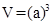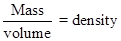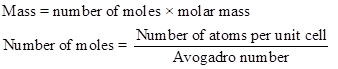Chapter 12, Problem 63IL

Chapter
Section
Textbook Problem

Potassium bromide has the same lattice structure as NaCl. Given the ionic radii of K+ (133 pm) and Br− (196 pm), calculate the density of KBr.

Interpretation Introduction

Interpretation:

The density of potassium bromide has to be calculated.

Concept introduction:

The volume of the atom is given below,The density of the unit cell is calculated as follows,Explanation

Still sussing out bartleby?

Check out a sample textbook solution.

See a sample solution

The Solution to Your Study Problems

Bartleby provides explanations to thousands of textbook problems written by our experts, many with advanced degrees!

Get Started

What is the definition of science?

An Introduction to Physical Science

Why might you say that atoms are mostly empty space?

Horizons: Exploring the Universe (MindTap Course List)

What characteristics indicate that amino acids exist as zwitterions?

Chemistry for Today: General, Organic, and Biochemistry